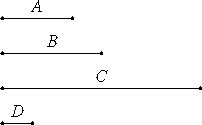# Proposition 31

If an odd number is relatively prime to any number, then it is also relatively prime to double it.

Let the odd number A be relatively prime to any number B, and let C be double of B.

I say that A is relatively prime to C.If they are not relatively prime, then some number will measure them.

Let a number D measure them.

Now A is odd, therefore D is also odd. And since D which is odd measures C, and C is even, therefore D measures the half of C also.

But B is half of C, therefore D measures B. But it also measures A, therefore D measures A and B which are relatively prime, which is impossible.

Therefore A cannot but be relatively prime to C. Therefore A and C are relatively prime.

Therefore, if an odd number is relatively prime to any number, then it is also relatively prime to double it.

Q.E.D.

## Guide

A generalization of this proposition would be “If two numbers (2 and B in this proposition) are relatively prime to to any number (A), then their product (2B) is also relatively prime to it (A).” That is proposition VII.24.